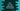# Dart for loop with example# for loop in Dart :

for loop is used to execute a piece of code for a specific amount of time. This amount of time is defined by a condition. It will keep executing the code until the condition is true.

for loop can also be used for iteration. You can iterate through a set of values like list, set, characters of a string etc. using a for loop.

In this tutorial, we will learn how to use dart for loop with examples.

## Syntax of for loop :

The syntax of a for loop is as below :

``````for(initialization, exit_condition, loop_end_step){
// code to execute
}``````

Here, initialization: It is the initialization part. Normally, one variable is created with an initial value. This part is executed at the start of the loop and only for once. exitcondition: This is a condition to check at the end of each iteration of the loop. The next iteration of the loop will start only if this condition is _true. This condition is linked to the variable that is created on the first initialization step. loopendstep: This is the step to execute at the end of each iteartion. This step is used to change the value of the variable.

For example :

``````main() {
for (var i = 0; i < 10; i++) {
print("i = \$i");
}
}``````

It will print the value of i on each iteration.

Here, var i = 0 is the initialization step. It initialized one variable i with 0 as its value.

i < 10 is the exit condition. This loop will run until this condition is true.

i++ is loop end step. This part will run at the end of each execution of the loop.

So, this loop will run from i = 0 to i = 9 with 1 increment on each step.

### for loop with multiple variables :

We can run one for loop with more than one variables. Initialize these variables by separating them with comma and change them in the loop end step :

``````main() {
for (var i = 0, j = 1, k = 2; i < 10; i = j, j = k, k++) {
print("i = \${i}, j = \${j}, k = \${k}");
}
}``````

Here, we have three variables with three different end conditions. This loop will run until the value of i is less than 10.

It will print the below output :

``````i = 0, j = 1, k = 2
i = 1, j = 2, k = 3
i = 2, j = 3, k = 4
i = 3, j = 4, k = 5
i = 4, j = 5, k = 6
i = 5, j = 6, k = 7
i = 6, j = 7, k = 8
i = 7, j = 8, k = 9
i = 8, j = 9, k = 10
i = 9, j = 10, k = 11``````

### Nested loop :

If we run one for loop inside another, it is called nested for loop. For example :

``````main() {
for (var i = 0; i < 3; i++) {
for (var j = 0; j < 3; j++) {
print("i = \${i}, j = \${j}");
}
}
}``````

This will print the below output :

``````i = 0, j = 0
i = 0, j = 1
i = 0, j = 2
i = 1, j = 0
i = 1, j = 1
i = 1, j = 2
i = 2, j = 0
i = 2, j = 1
i = 2, j = 2``````

For each iteration of the outer loop, the inner loop will run once. Inner loop increases the execution time. You can have infinite number of inner loops.